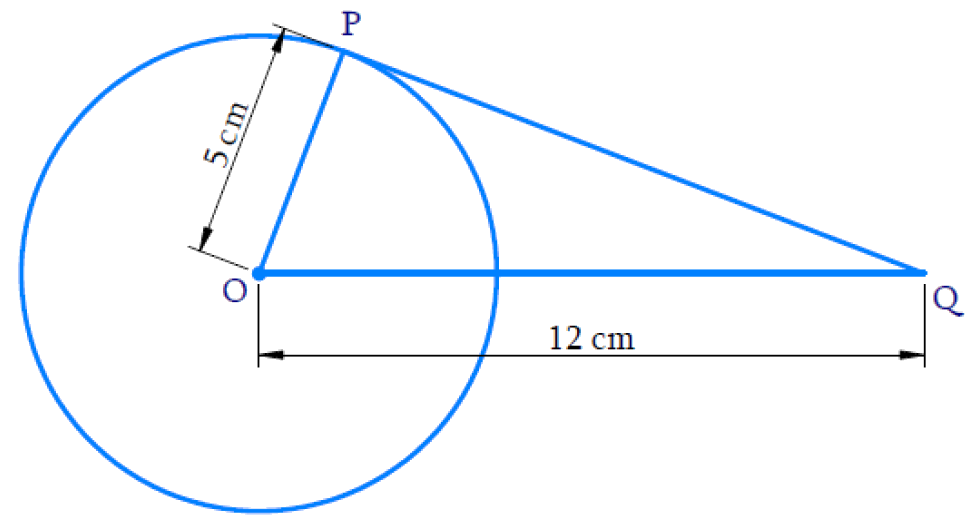# Ex.10.1 Q3. Circles Solution - NCERT Maths Class 10

Go back to  'Ex.10.1'

## Question

A tangent $$PQ$$ at a point $$P$$ of a circle of radius $$\text{5 cm}$$ meets a line through the center $$O$$ at a point $$Q$$ so that $$OQ = \text{12 cm.}$$ Length $$PQ$$ is:

(A) $$\text{12 cm}$$

(B) $$\text{13 cm}$$

(C) $$\text{8.5 cm}$$

(D) $$\sqrt{119} \,\rm{cm.}$$

## Text Solution

What is Known?

Radius $$OP = \text{5 cm}$$

$$OQ = \text{12 cm}$$What is Unknown?

Length of the tangent $$PQ$$

Reasoning:

$$\Delta {OPQ}$$ is a right-angle triangle according to Theorem $$10.1 :$$ The tangent at any point of a circle is perpendicular to the radius through the point of contact.

Steps:

By Pythagoras theorem

\begin{align} {OQ} ^ { 2 } & = {O P} ^ { 2 } + {P Q} ^ { 2 } \\ 12 ^ { 2 } & = 5 ^ { 2 } + {P Q} ^ { 2 } \\ 144 & = 25 + {P Q} ^ { 2 } \\ {P Q} ^ { 2 } & = 119 \\ {P Q} & = \sqrt { 119 } \rm {\;cm } \end{align}